# SVM Tutorial: How to classify text in R

In this tutorial I will show you how to classify text with SVM in R.The main steps to classify text in R are:

1. Create a new RStudio project
2. Install the required packages
3. Read the data
4. Prepare the data
5. Create and train the SVM model
6. Predict with new data

## Step 1: Create a new RStudio Project

To begin with, you will need to download and install the RStudio development environment.

Once you installed it, you can create a new project by clicking on "Project: (None)" at the top right of the screen :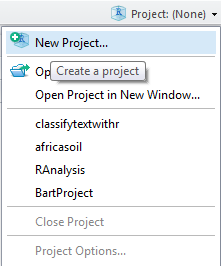Create a new project in R Studio

This will open the following wizard, which is pretty straightforward: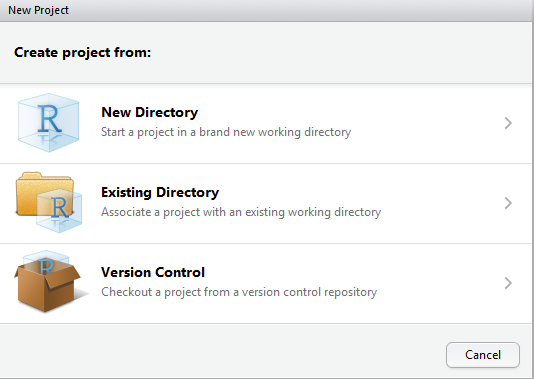Select "New Directory"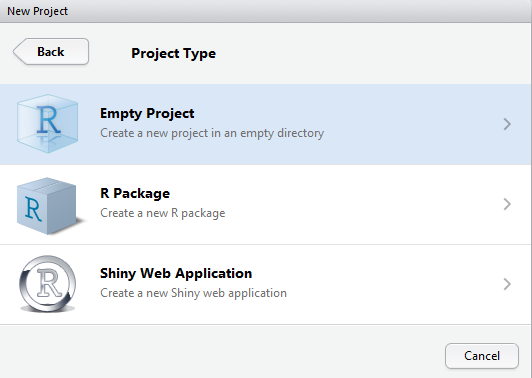We will create an empty project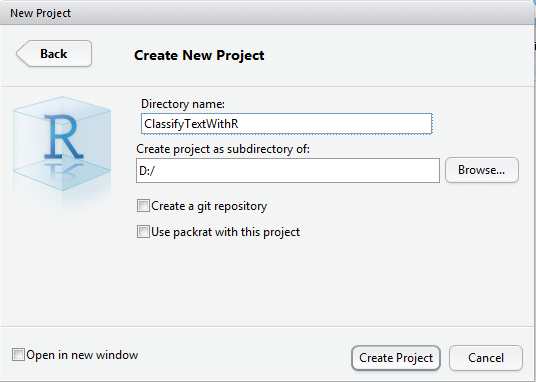Name your project and you are done

Now that the project is created, we will add a new R Script: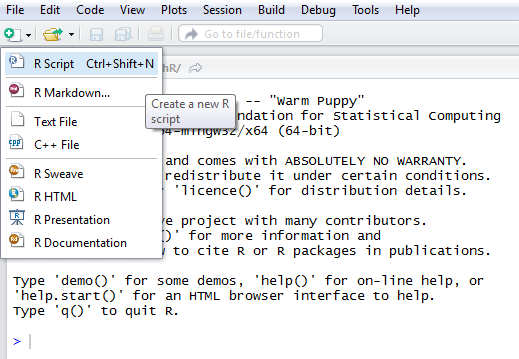You can save this script, by giving the name you wish, for instance "Main"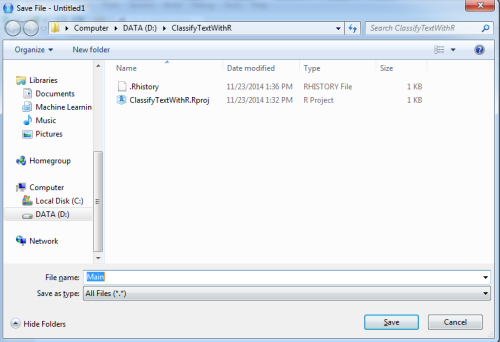Saving our first script

## Step 2: Install the required packages

To easily classify text with SVM,  we will use the RTextTools package.

In RStudio, on the right side, you can see a tab named "Packages", select id and then click "Install R packages"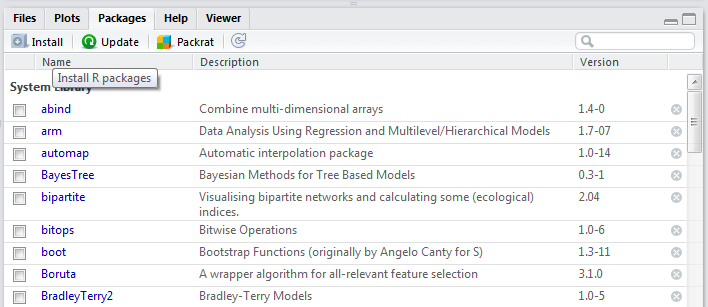RStudio list all installed packages

This will open a popup, you now need to enter the name of the package RTextTools.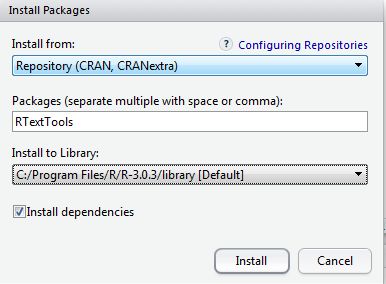Be sure to check "Install dependencies"

Once it is installed, it will appear on the package list. Check it to load it in the environment.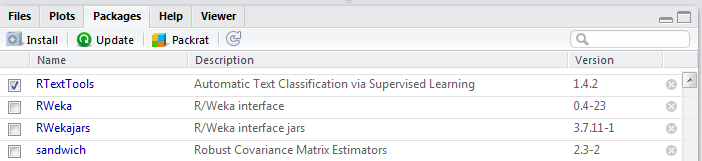The RTextTools package now appears on the list

## Step 3: Read the data

For this tutorial we will use a very simple data set (click to download).
With just a few lines of R, we load the data in memory:

``` # Load the data from the csv file
dataDirectory <- "D:/" # put your own folder here
data <- read.csv(paste(dataDirectory, 'sunnyData.csv', sep=""), header = TRUE)
```

## Step 4: Prepare the data

The data has two columns: Text and IsSunny.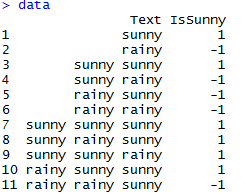Our simple data set

We will need to convert it to a Document Term Matrix.

To understand what a document term matrix is or to learn more about the data set, you can read:   How to prepare your data for text classification ?

### Using RTextTools

The RTextTools package provides a powerful way to generate document term matrix with the create_matrix function:

```# Create the document term matrix
dtMatrix <- create_matrix(data["Text"])
```

Typing the name of the matrix in the console, shows us some interesting facts :For instance, the sparsity can help us decide whether we should use a linear kernel.

## Step 5: Create and train the SVM model

In order to train a SVM model with RTextTools, we need to put the document term matrix inside a container. In the container's configuration, we indicate that the whole data set will be the training set.

```# Configure the training data
container <- create_container(dtMatrix, data\$IsSunny, trainSize=1:11, virgin=FALSE)

# train a SVM Model
model <- train_model(container, "SVM", kernel="linear", cost=1)
```

The code above trains a new SVM model with a linear kernel.

Note:

• both the create_container and train_model  methods are RTextTools methods.
Under the hood, RTextTools uses the e1071 package which is a R wrapper around libsvm;
• the virgin=FALSE argument is here to tell RTextTools not to save an analytics_virgin-class object inside the container. This parameter does not interest us now but is required by the function.

## Step 6: Predict with new data

Now that our model is trained, we can use it to make new predictions !

We will create new sentences which were not in the training data:

```# new data
predictionData <- list("sunny sunny sunny rainy rainy", "rainy sunny rainy rainy", "hello", "", "this is another rainy world")
```

Before continuing, let's check the new sentences :

• "sunny sunny sunny rainy rainy"
This sentence talks more about the sunny weather than the rainy. We expect it to be classified as sunny (+1).
• "rainy sunny rainy rainy"
This sentence talks more about the rainy weather than the sunny. We expect it to be classified as rainy (-1).
• ""
This sentence has no word, it should return either +1 or -1 in function of the decision boundary.
• "hello"
This sentence has a word which was not present in the training set. It will be equivalent to ""
• "this is another rainy world"
This sentence has several worlds not in the training set, and the word rainy. It is equivalent to the sentence "rainy" and should be classified "-1"

We create a document term matrix for the test data:

```# create a prediction document term matrix
predMatrix <- create_matrix(predictionData, originalMatrix=dtMatrix)
```

Notice that this time we provided the originalMatrix as a parameter. This is because we want the new matrix to use the same vocabulary as the training matrix.
Without this indication, the function will create a document term matrix using all the words of the test data (rainy, sunny, hello, this, is, another, world). It means that each sentence will be represented by a vector containing 7 values (one for each word) !

Such a matrix won't be compatible with the model we trained earlier because it expect vectors containing 2 values (one for rainy, one for sunny).

We now create the container:

```# create the corresponding container
predSize = length(predictionData);
predictionContainer <- create_container(predMatrix, labels=rep(0,predSize), testSize=1:predSize, virgin=FALSE)
```

Two things are different:

• we use a zero vector for labels, because we want to predict them
• we specified testSize instead of trainingSize so that the data will be used for testing

Eventually, we can make predictions:

```# predict
results <- classify_model(predictionContainer, model)
results
```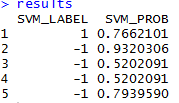Predictions and their probabilities

As expected the first sentence has been classified as sunny and the second and last one as rainy.
We can also see that the third and fourth sentences  ("hello" and "") have been classified as rainy, but the probability is only  52% which means our model is not very confident on these two predictions.

## Summary of this SVM Tutorial

Congratulations ! You have trained a SVM model and used it to make prediction on unknown data. We only saw a bit of what is possible to do with RTextTools.

If you are interested by learning how to classify text with other languages you can read:

You can also get all the code from this article:

### 99 thoughts on “SVM Tutorial: How to classify text in R”

1.Thanks for this tutorial, When i tried to execute the script I got the below error
Error in if (attr(weighting, "Acronym") == "tf-idf") weight <- 1e-09 :
argument is of length zero
As per the comments I tried below code and worked perfectly
trace(create_matrix,edit=T) and then replaced 'Acronym' with 'acronym',
But, now the problem is while executing from command prompt I'm getting the above error again, also on closing and opening R, the create matrix changes to default. I'm looking for permanent solution to fix this bug. Can anyone help?

Reply
2.excellent explanation!!

Reply
3.This is very helpful. Thanks a lot. I have some doubts.How can we create confusion matrix for this and how to find accuracy?

Reply